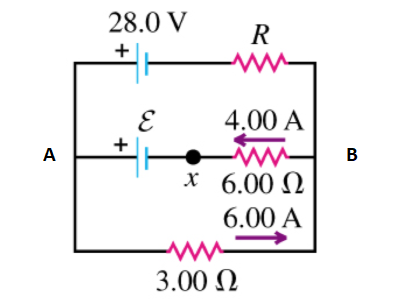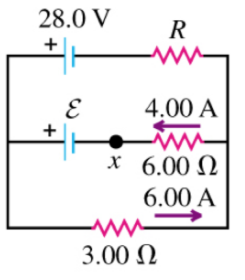# Problem: In the circuit shown in the figure (Figure 1) find(a) the current in resistor R(b) the resistance R(c) The unknown emf ℰ(d) If the circuit is broken at point x, what is the current in resistor R?

###### FREE Expert Solution(a) At Junction B, the sum of currents entering the junction is equal to the sum of currents leaving the junction.

ΣCurrents in = ΣCurrent out

6 A = 4 A + IR

IR = 2A

Current through resistor R is 2A

86% (216 ratings)###### Problem Details

In the circuit shown in the figure (Figure 1) find(a) the current in resistor R

(b) the resistance R

(c) The unknown emf ℰ

(d) If the circuit is broken at point x, what is the current in resistor R?

Frequently Asked Questions

What scientific concept do you need to know in order to solve this problem?

Our tutors have indicated that to solve this problem you will need to apply the Kirchhoff's Loop Rule concept. You can view video lessons to learn Kirchhoff's Loop Rule. Or if you need more Kirchhoff's Loop Rule practice, you can also practice Kirchhoff's Loop Rule practice problems.

What professor is this problem relevant for?

Based on our data, we think this problem is relevant for Professor Velissaris' class at UCF.